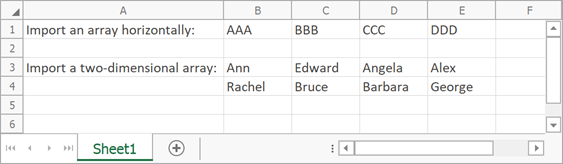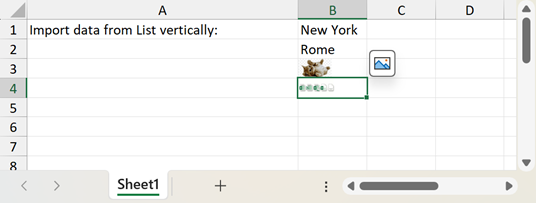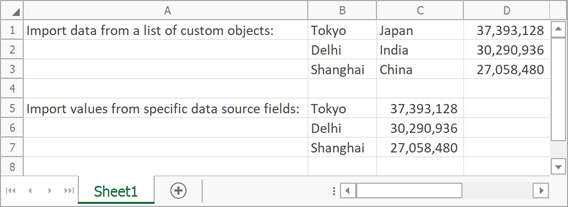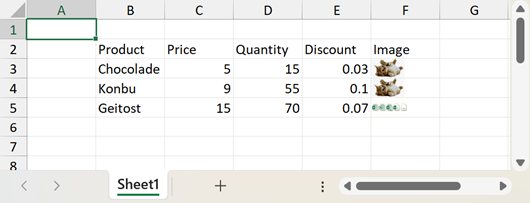.NET Framework 4.5.2+

# How to: Import Data to a Worksheet

You can import data to worksheet cells from different data sources (for example, arrays, lists, and data tables). To import data into a worksheet, call the WorksheetExtensions.Import method and pass the following parameters:

• An object that specifies a data source;
• Row and column indexes of the start cell in the destination cell range;
• A Boolean value that specifies whether to insert data vertically or horizontally. Use this parameter when you import data from a one-dimensional array or list.
• A DataSourceImportOptions instance that contains import options (optional).

Important

The WorksheetExtensions class is defined in DevExpress.Docs.v23.1.dll. Add this assembly to your project to use worksheet extensions. You need a license for the DevExpress Office File API Subscription or DevExpress Universal Subscription to use this assembly in production code.

## Import Data from Arrays

``````using DevExpress.Spreadsheet;
using System;
// ...

Worksheet worksheet = workbook.Worksheets;
// Create an array containing string values.
string[] array = new string[] { "AAA", "BBB", "CCC", "DDD" };

// Import the array into the worksheet and insert it horizontally, starting with the B1 cell.
worksheet.Import(array, 0, 1, false);

// Create a two-dimensional array containing string values.
String[,] names = new String[2, 4]{
{"Ann", "Edward", "Angela", "Alex"},
{"Rachel", "Bruce", "Barbara", "George"}
};

// Import the two-dimensional array into the worksheet and insert it, starting with the B3 cell.
worksheet.Import(names, 2, 1);
``````

The following image shows the result:## Import Data from a List

``````using DevExpress.Spreadsheet;
using System.Collections.Generic;
//...

Worksheet worksheet = workbook.Worksheets;

// Create a list that contains string values.
List<string> cities = new List<string>();

// Insert list values into the worksheet vertically.
// Data starts with the B1 cell.
worksheet.Import(cities, 0, 1, true);
``````

The following image shows the result:## Import Data from a List of Custom Objects

``````using DevExpress.Spreadsheet;
using System.Collections.Generic;
// ...

void ImportListValues(Workbook workbook) {
Worksheet worksheet = workbook.Worksheets;

// Create a list of the most populated cities.
var cities = new List<City>()
{
new City(){ Name = "Tokyo", Country= "Japan", Population = 37393128 },
new City(){ Name = "Delhi", Country= "India", Population = 30290936 },
new City(){ Name = "Shanghai", Country= "China", Population = 27058480},
};

// Insert list values into the worksheet.
// Data starts with the B1 cell.
worksheet.Import(cities, 0, 1);
// Apply the number format to the "Population" column.
worksheet["D1:D3"].NumberFormat = "_(* #,##0_);_(* (#,##0);_(* \" - \"??_);_(@_)";

// Import values from specific data source fields.
// Data starts with the B5 cell.
var importOptions = new DataSourceImportOptions()
{
PropertyNames = new string[] { "Name", "Population" }
};
worksheet.Import(cities, 4, 1, importOptions);
// Apply the number format to the "Population" column.
worksheet["C5:C7"].NumberFormat = "_(* #,##0_);_(* (#,##0);_(* \" - \"??_);_(@_)";
}

class City
{
public string Name { get; set; }
public string Country { get; set; }
public int Population { get; set; }
}
``````

The following image shows the result:## Import Data from a Data Table

The following code illustrates how to import data from a DataTable object to a worksheet. Note that the cell data types are set automatically, according to the data types of the source column. Cell formats are set automatically to the default value for the cell data type. However, you can easily change them, as described in the How to: Specify Number or Date Format for Cell Content topic.

``````Private Sub ImportDataTable()

Dim worksheet As Worksheet = spreadsheetControl1.Document.Worksheets(0)

' Create a "Products" DataTable object with four columns.
Dim sourceTable As New DataTable("Products")

' Import data from the data table into the worksheet and insert it, starting with the B2 cell.
worksheet.Import(sourceTable, True, 1, 1)
End Sub
``````

The following image shows the result:Tip

You can also use the following method to import data into a worksheet:

1. Bind the worksheet to a read-only data source as described in this help topic: Data Binding.

2. Call the WorksheetDataBindingCollection.Remove or WorksheetDataBindingCollection.Clear method to remove the binding.